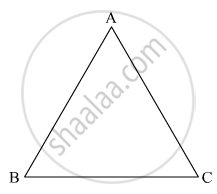PUC Karnataka Science Class 12Department of Pre-University Education, Karnataka
Share

# Find the angle of incidence at face AB so that the emergent ray grazes along the face AC. - PUC Karnataka Science Class 12 - Physics

#### Question

Find the angle of incidence at face AB so that the emergent ray grazes along the face AC.#### Solution

from snell's law we know that
sini/sinr =μ_(12)

For the emergent ray to graze at the face AC, the angle of refraction should be 90°.

So, applying snell's law at face AC, we get (sini_(AC)/sinr_(AC) )= μ_(21)

⇒ (sin i_(AC)/sin 90^@)=1/sqrt2 or i_(AC)= 45°.

From geometry we can see that angle of refraction at face AB is 15°.

So applying snell's law we get (sin i_(AB)/sin r_(AB))= μ_(12)

or sin i_(AB) = sin r_(AB)×μ_(12)

or i_(AB) =sin^-1(sqrt2sin15°)

Is there an error in this question or solution?

#### APPEARS IN

Solution Find the angle of incidence at face AB so that the emergent ray grazes along the face AC. Concept: Refraction Through a Prism.
S